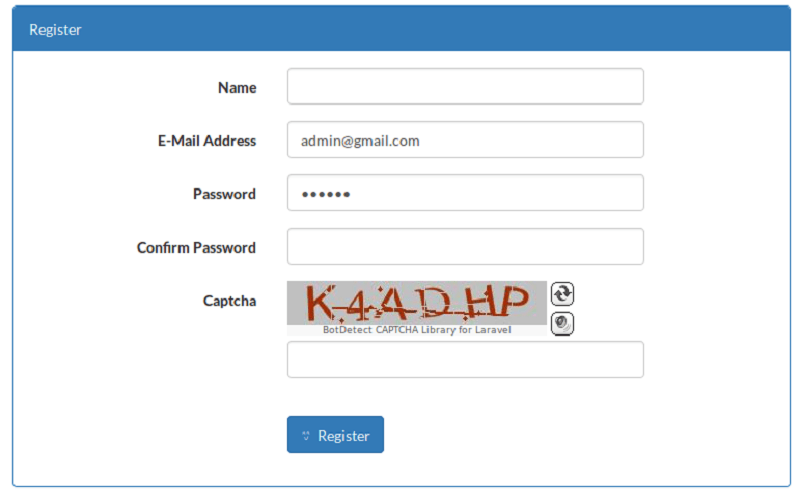# FahmidasClassroom

Learn by easy stepsThis tutorials shows a very simple way of developing captcha by using html and javascript code. You have to create some images with png extension that contains some characters without any order to test the following code.

Steps:

1. Create some images for using as captcha text.

``````
<script language="javascript">
var imgarray = ["GRYX","MDAO","PKHJ","FQUV","SKHW"];
var rimg = new Image();
var index;

function RandomImage()
{
index = Math.random();
index = Math.floor(index*10);
var l = imgarray.length-1;
if(index > l) index= index%l;
rimg.src = "Images/"+index+".png";
document.vimg.src= rimg.src;

}
function Check()
{
var val = document.v.t.value;
var match_t = false;
for(var i=0; i<imgarray.length; i++)
{
if(val == imgarray[i] && (i == index))
{
match_t = true;
break;
}
}
if(match_t == false)
else
}

function Check_key(e)
{
if (e.keyCode == 13) {
Check();

}

}

</script>
``````

3. Add the following code with body tag.

``````